# 1 Introduction

The aim of cartography is to obtain thematic maps with the visual quality of those build with a classical mapping or GIS software.

Users of the package could belong to one of two categories: cartographers willing to use R or R users willing to create maps. Therefore, its functions have to be intuitive to cartographers and ensure compatibility with common R workflows.

cartography uses sf or sp objects to produce base graphics. As most of the internals of the package relies on sf functionalities, the preferred format for spatial objects is sf.

# 2 Features

cartography’s functions can be classified in the following categories :

• Symbology
Each function focuses on a single cartographic representation (e.g. proportional symbols or choropleth representation) and displays it on a georeferenced plot. This solution allows to consider each representation as a layer and to overlay multiple representations on a same map.
Each function has two main arguments that are:

• x, a spatial object (preferably an sf object),
• var, the name of a variable to map.

sp objects are handled through the spdf argument if the variable is contained within the Spatial*DataFrame and through spdf, spdfid, df, dfid if the variable is in a separate data.frame that needs to be joined to the Spatial*DataFrame.

Many parameters are available to fine tune the cartographic representations. These parameters are the common ones found in GIS and automatic cartography tools (e.g. classification and color palettes used in choropleth maps, symbols sizes used in proportional symbols maps…).

• Transformations
A set of functions is dedicated to the creation or transformation of spatial objects (e.g. borders extraction, grid or links creation). These functions are provided to ease the creation of some more advanced maps that usually need geo-processing.

• Map Layout
Along with the cartographic functions, some other functions are dedicated to layout design (e.g. customizable scale bar, north arrow, title, sources or author information…).

• Color Palettes
16 original color palettes are shipped within the package. Those palettes can be customized and combined.

• Legends
Legends are displayed by default along cartographic layers but more parameters are available through legend*() functions.

• Classification
getBreaks() give access to most of the classification methods used for data binning.

# 3 Examples of thematic maps

## 3.1 Proportional Symbols

propSymbolsLayer() displays symbols with areas proportional to a quantitative variable (stocks). Several symbols are available (circles, squares, bars). The inches argument is used to customize the symbols sizes.

library(sf)
library(cartography)
# path to the geopackage file embedded in cartography
path_to_gpkg <- system.file("gpkg/mtq.gpkg", package="cartography")
# import to an sf object
mtq <- st_read(dsn = path_to_gpkg, quiet = TRUE)
# plot municipalities (only borders are plotted)
plot(st_geometry(mtq), col = "grey80", border = "grey")
# plot population
propSymbolsLayer(
x = mtq,
var = "POP",
inches = 0.25,
col = "brown4",
legend.pos = "topright",
legend.title.txt = "Total population"
)
# layout
layoutLayer(title = "Population Distribution in Martinique",
sources = "Sources: Insee and IGN, 2018",
author = paste0("cartography ", packageVersion("cartography")),
frame = FALSE, north = FALSE, tabtitle = TRUE)
# north arrow
north(pos = "topleft")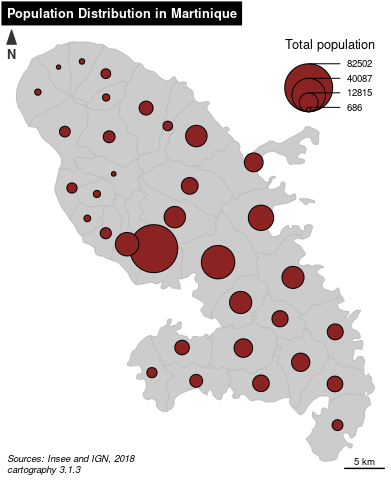## 3.2 Choropleth Map

In choropleth maps, areas are shaded according to the variation of a quantitative variable. They are used to represent ratios or indices.

choroLayer() displays choropleth maps . Arguments nclass, method and breaks allow to customize the variable classification. getBreaks() allow to classify outside of the function itself. Colors palettes are defined with col and a set of colors can be created with carto.pal() (see also display.carto.all()).

library(sf)
library(cartography)
# path to the geopackage file embedded in cartography
path_to_gpkg <- system.file("gpkg/mtq.gpkg", package="cartography")
# import to an sf object
mtq <- st_read(dsn = path_to_gpkg, quiet = TRUE)
# population density (inhab./km2) using sf::st_area()
mtq$POPDENS <- 1e6 * mtq$POP / st_area(mtq)
# plot municipalities (only the backgroung color is plotted)
plot(st_geometry(mtq), col = NA, border = NA, bg = "#aadaff")
# plot population density
choroLayer(
x = mtq,
var = "POPDENS",
method = "geom",
nclass=5,
col = carto.pal(pal1 = "sand.pal", n1 = 5),
border = "white",
lwd = 0.5,
legend.pos = "topright",
legend.title.txt = "Population Density\n(people per km2)",
)
# layout
layoutLayer(title = "Population Distribution in Martinique",
sources = "Sources: Insee and IGN, 2018",
author = paste0("cartography ", packageVersion("cartography")),
frame = FALSE, north = FALSE, tabtitle = TRUE, theme= "sand.pal")
# north arrow
north(pos = "topleft")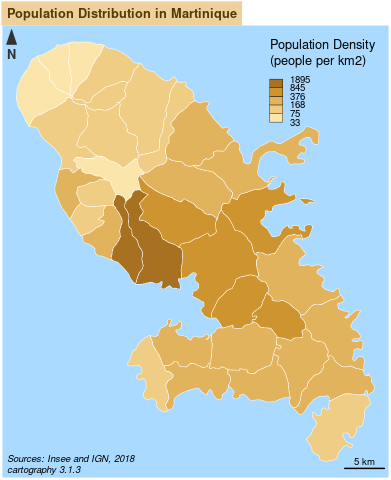## 3.3 Colored Pencil and Typologies Map

getPencilLayer() transforms POLYGONS or MULTIPOLYGONS in MULTILINESTRINGS. This function creates a layer that mimicks a pencil hand-drawing.

typoLayer() displays a typology map of a qualitative variable. legend.values.order is used to set the modalities order in the legend.

library(sf)
library(cartography)
# path to the geopackage file embedded in cartography
path_to_gpkg <- system.file("gpkg/mtq.gpkg", package="cartography")
# import to an sf object
mtq <- st_read(dsn = path_to_gpkg, quiet = TRUE)
# transform municipality multipolygons to (multi)linestrings
mtq_pencil <- getPencilLayer(
x = mtq,
size = 400,
lefthanded = F
)
# plot municipalities (only the backgroung color is plotted)
plot(st_geometry(mtq), col = "white", border = NA, bg = "lightblue1")
typoLayer(
x = mtq_pencil,
var="STATUS",
col = c("aquamarine4", "yellow3","wheat"),
lwd = .7,
legend.values.order = c("Prefecture",
"Sub-prefecture",
"Simple municipality"),
legend.pos = "topright",
legend.title.txt = "",
)
#  plot municipalities
plot(st_geometry(mtq), lwd = 0.5, border = "grey20", add = TRUE, lty = 3)
# labels for a few  municipalities
labelLayer(x = mtq[mtq$STATUS != "Simple municipality",], txt = "LIBGEO", cex = 0.9, halo = TRUE, r = 0.15) # title, source, author layoutLayer(title = "Administrative Status", sources = "Sources: Insee and IGN, 2018", author = paste0("cartography ", packageVersion("cartography")), north = FALSE, tabtitle = TRUE, postitle = "right", col = "white", coltitle = "black") # north arrow north(pos = "topleft")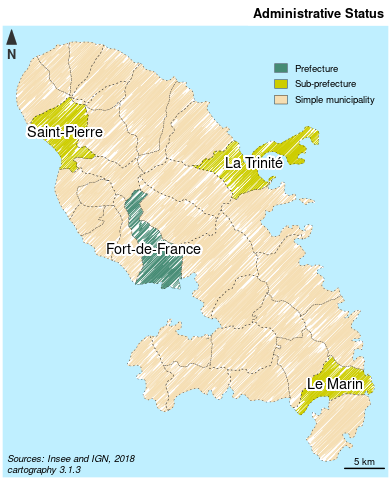## 3.4 Proportional Symbols and Choropleth Map propSymbolsChoroLayer() creates a map of symbols that are proportional to values of a first variable and colored to reflect the classification of a second variable. A combination of propSymbolsLayer() and choroLayer() arguments is used. library(sf) library(cartography) # path to the geopackage file embedded in cartography path_to_gpkg <- system.file("gpkg/mtq.gpkg", package="cartography") # import to an sf object mtq <- st_read(dsn = path_to_gpkg, quiet = TRUE) # Plot the municipalities plot(st_geometry(mtq), col="darkseagreen3", border="darkseagreen4", bg = "lightblue1", lwd = 0.5) # Plot symbols with choropleth coloration propSymbolsChoroLayer( x = mtq, var = "POP", border = "grey50", lwd = 1, legend.var.pos = "topright", legend.var.title.txt = "Population", var2 = "MED", method = "equal", nclass = 4, col = carto.pal(pal1 = "sand.pal", n1 = 4), legend.var2.values.rnd = -2, legend.var2.pos = "left", legend.var2.title.txt = "Median\nIncome\n(in euros)" ) # layout layoutLayer(title="Population & Wealth in Martinique, 2015", author = "cartography 2.1.3", sources = "Sources: Insee and IGN, 2018", scale = 5, tabtitle = TRUE, frame = FALSE) # north arrow north(pos = "topleft")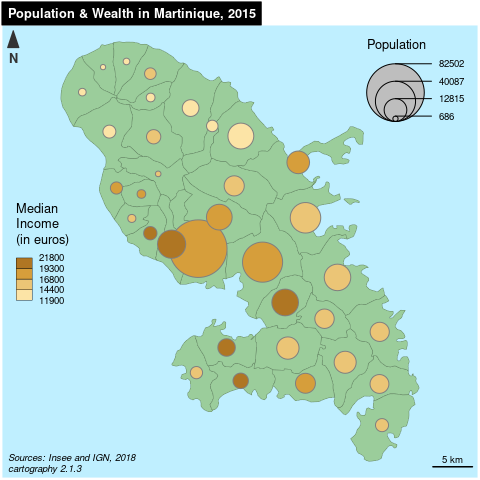## 3.5 Proportional Symbols and Typology Map propSymbolsTypoLayer() creates a map of symbols that are proportional to values of a first variable and colored to reflect the modalities of a second qualitatice variable. A combination of propSymbolsLayer() and typoLayer() arguments is used. library(sf) library(cartography) # path to the geopackage file embedded in cartography path_to_gpkg <- system.file("gpkg/mtq.gpkg", package="cartography") # import to an sf object mtq <- st_read(dsn = path_to_gpkg, quiet = TRUE) # Plot the municipalities plot(st_geometry(mtq), col="#f2efe9", border="#b38e43", bg = "#aad3df", lwd = 0.5) # Plot symbols with choropleth coloration propSymbolsTypoLayer( x = mtq, var = "POP", inches = 0.5, symbols = "square", border = "white", lwd = .5, legend.var.pos = "topright", legend.var.title.txt = "Population", var2 = "STATUS", legend.var2.values.order = c("Prefecture", "Sub-prefecture", "Simple municipality"), col = carto.pal(pal1 = "multi.pal", n1 = 3), legend.var2.pos = c(692000, 1607000), legend.var2.title.txt = "Administrative\nStatus" ) # layout layoutLayer(title="Population Distribution in Martinique", author = "cartography 2.1.3", sources = "Sources: Insee and IGN, 2018", scale = 5, tabtitle = TRUE, frame = FALSE) # north arrow north(pos = "topleft")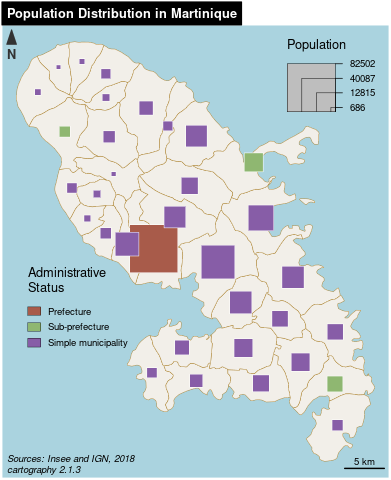## 3.6 Label Map labelLayer() is dedicated to the display of labels on a map. The overlap = FALSE argument displays non overlapping labels. library(sf) library(cartography) # path to the geopackage file embedded in cartography path_to_gpkg <- system.file("gpkg/mtq.gpkg", package="cartography") # import to an sf object mtq <- st_read(dsn = path_to_gpkg, quiet = TRUE) # plot municipalities plot(st_geometry(mtq), col = "#e4e9de", border = "darkseagreen4", bg = "lightblue1", lwd = 0.5) # plot labels labelLayer( x = mtq, txt = "LIBGEO", col= "black", cex = 0.7, font = 4, halo = TRUE, bg = "white", r = 0.1, overlap = FALSE, show.lines = FALSE ) # map layout layoutLayer( title = "Municipalities of Martinique", sources = "Sources: Insee and IGN, 2018", author = paste0("cartography ", packageVersion("cartography")), frame = FALSE, north = TRUE, tabtitle = TRUE, theme = "taupe.pal" )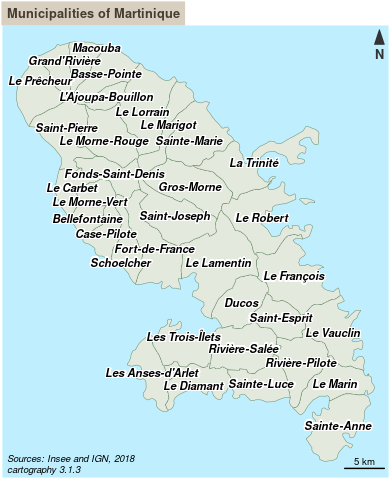## 3.8 Isopleth Map smoothLayer() is deprecated. See the package potential instead. Isopleth maps are based on the assumption that the phenomenon to be represented has a continuous distribution. These maps use a spatial interaction modeling approach which aims to compute indicators based on stock values weighted by distance. It allows a spatial representation of the phenomenon independent from the initial heterogeneity of the territorial division. ## 3.9 Grid Map The grid-cell method is an option to overcome the arbitrariness and irregularity of an administrative division. It highlights the main trends in the data spatial distribution, splitting the territory in regular blocks. Statistical values are distributed over a regular grid. Cell values are classified and then displayed in areas of color. The principle adopted here is to set each cell’s value with a proportion of the initial geometrical units it overlay (share of intersected area). getGridLayer() builds a regular grid (squares or hexagons) based on a spatial object and computes data that match the grid layer. choroLayer() is then used to display the grid on a choropleth map. library(sf) library(cartography) # path to the geopackage file embedded in cartography path_to_gpkg <- system.file("gpkg/mtq.gpkg", package="cartography") # import to an sf object mtq <- st_read(dsn = path_to_gpkg, quiet = TRUE) # Create a grid layer, cell size area match the median municipality area mygrid <- getGridLayer( x = mtq, cellsize = median(as.numeric(st_area(mtq))), var = "POP", type = "hexagonal" ) # Compute population density in people per km2 mygrid$POPDENS <- 1e6 * mygrid$POP / mygrid$gridarea
# plot municipalities (only the backgroung color is plotted)
plot(st_geometry(mtq), col = NA, border = NA, bg = "#deffff")
# Plot the population density
choroLayer(x = mygrid, var = "POPDENS", method = "geom", nclass=5,
col = carto.pal(pal1 = "turquoise.pal", n1 = 5), border = "grey80",
lwd = 0.5, legend.pos = "bottomleftextra", add = TRUE,
legend.title.txt = "Population Density\n(people per km2)")
layoutLayer(title = "Population Distribution in Martinique",
sources = "Sources: Insee and IGN, 2018",
author = paste0("cartography ", packageVersion("cartography")),
frame = FALSE, north = FALSE, tabtitle = TRUE,
theme = "turquoise.pal")
# north arrow
north(pos = "topleft")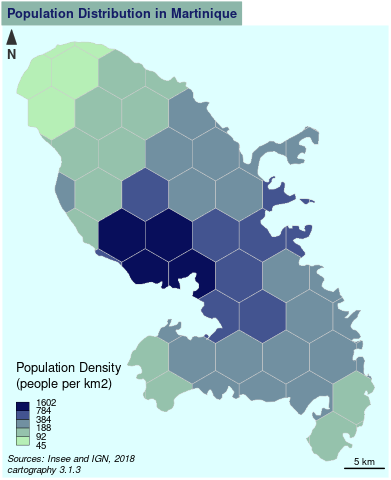## 3.10 Discontinuities Map

Discontinuities maps are based on the variation of a phenomena between contiguous units. This kind of representation focuses spatial breaks. The discontinuity intensity is expressed by the borders’ thickness.
getBorders() is used to build a spatial object of borders between units. Each resulting borders contains the ids of its two neighboring units. It is possible to complement these borders with getOuterBorders() to compute borders between non-contiguous units (e.g. maritime borders). discLayer() computes and displays discontinuities, lines widths reflect the ratio or the absolute difference between values of an indicator in two neighboring units.

library(sf)
library(cartography)
# path to the geopackage file embedded in cartography
path_to_gpkg <- system.file("gpkg/mtq.gpkg", package="cartography")
# import to an sf object
mtq <- st_read(dsn = path_to_gpkg, quiet = TRUE)
# Compute the population density (inhab./km2) using sf::st_area()
mtq$POPDENS <- as.numeric(1e6 * mtq$POP / st_area(mtq))
# Get a SpatialLinesDataFrame of countries borders
mtq.contig <- getBorders(mtq)
# plot municipalities (only the backgroung color is plotted)
plot(st_geometry(mtq), col = NA, border = NA, bg = "lightblue1",
xlim = c(690574, 745940))
# Plot the population density with custom breaks
choroLayer(x = mtq, var = "MED",
breaks = c(min(mtq$MED), seq(13000, 21000, 2000), max(mtq$MED)),
col = carto.pal("green.pal", 6),border = "white", lwd = 0.5,
legend.pos = "topright", legend.title.txt = "Median Income\n(euros)",
# Plot discontinuities
discLayer(
x = mtq.contig,
df = mtq,
var = "MED",
type = "rel",
method = "geom",
nclass = 3,
threshold = 0.4,
sizemin = 0.7,
sizemax = 6,
col = "red4",
legend.values.rnd = 1,
legend.title.txt = "Relative\nDiscontinuities",
legend.pos = "right",
)
# Layout
layoutLayer(title = "Wealth Disparities in Martinique, 2015",
author =  paste0("cartography ", packageVersion("cartography")),
sources = "Sources: Insee and IGN, 2018",
frame = FALSE, scale = 5, tabtitle = TRUE,theme = "grey.pal")
# north arrow
north(pos = "topleft")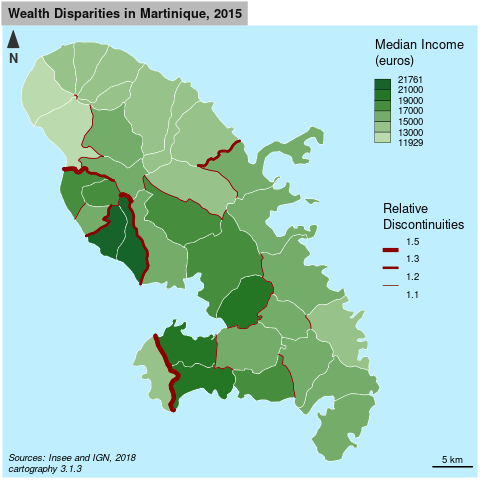## 3.11 Mapping sp Objects

SpatialPointsDataFrame and SpatialPolygonsDataFrame (from sp) are handled through the spdf argument if the variable is contained within the Spatial*DataFrame and through spdf, spdfid, df, dfid if the variable is in a separate data.frame that needs to be joined to the Spatial*DataFrame.

library(sp)
library(cartography)
data("nuts2006")
# Plot a layer with the extent of the EU28 countries with only a background color
plot(nuts0.spdf, border = NA, col = NA, bg = "#A6CAE0")
# Plot non european space
plot(world.spdf, col  = "#E3DEBF", border = NA, add = TRUE)
# Plot Nuts2 regions
plot(nuts0.spdf, col = "grey60",border = "white", lwd = 0.4, add = TRUE)
# plot the countries population
propSymbolsLayer(
spdf = nuts0.spdf,
df = nuts0.df,
spdfid = "id",
dfid = "id",
var = "pop2008",
legend.pos = "topright",
col = "red4",
border = "white",
legend.title.txt = "Population"
)
# layout
layoutLayer(title = "Population in Europe, 2008",
sources = "Data: Eurostat, 2008",
author =  paste0("cartography ", packageVersion("cartography")),
scale = 500, frame = TRUE, col = "#688994")
# north arrow
north("topleft")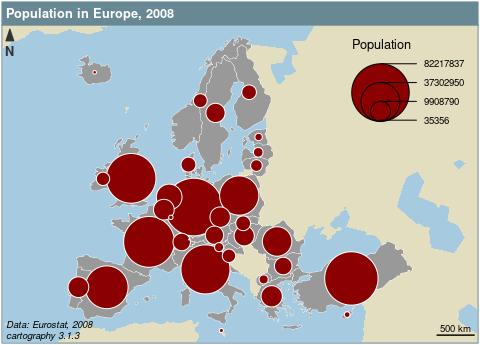# 4 Datasets

Several datasets are embedded in the package:

• A GeoPackage of Martinique municipalities can be imported via the st_read() function of the sf package.
• Sources: Base comparateur de territoires (upload date: 2018-09-25) & ADMIN EXPRESS-COG (2018 edition).
• Citation: Insee and IGN, 2018
• Fields:
• INSEE_COM: Municipality identifier
• LIBGEO: Municipality name
• POP: Total population, 2015
• MED: Median disposable income adjusted per equivalent household member, in euros, 2015
• CHOM: Unemployed population, 2015
• ACT: Active population, 2015
• A csv file of professional mobility flows between Martinique municipalities.
• Sources: Flux de mobilité - déplacements domicile-lieu de travail (upload date: 2018-08-01)
• Citation: Insee, 2018
• Fields:
• i: Municipality of residence identifier
• j: Municipality of workplace identifier
• fij: Flows of workers (employed population, 15 y.o. or more, 2015, only flows > 100)
• sj: Administrative status of the workplace municipality
• A set of sp objects and data.frames on European regions (NUTS) can be loaded in the environment via data(nuts2006). Each layer of this dataset is directly described in the documentation (e.g. ?nuts0.spdf).